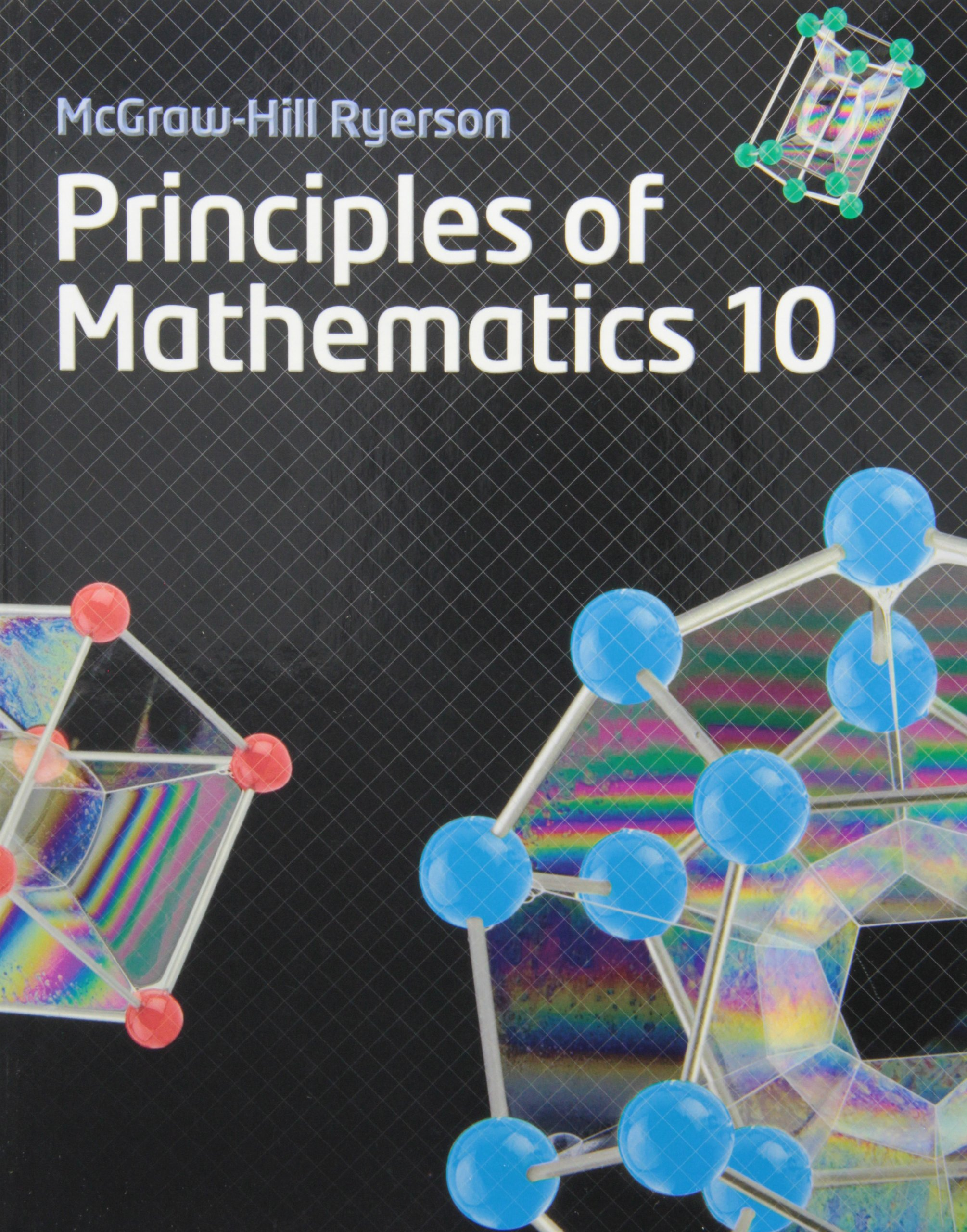Principles of Mathematics (Gr. 10 Academic - MPM2D)
This course enables students to broaden their understanding of relationships and extend their problem-solving and algebraic skills. Students will explore quadratic relations and their applications; solve and apply linear systems; verify properties of geometric figures using analytic geometry; and investigate the trigonometry of right and acute triangles. Students will reason mathematically and communicate their thinking as they solve multi-step problems.

Unit 1 - Analytic GeometryChapter 7&8 - Introduction to Trigonometry
 We are here:Ch. 7 - Intro to Trig! (I love trig)